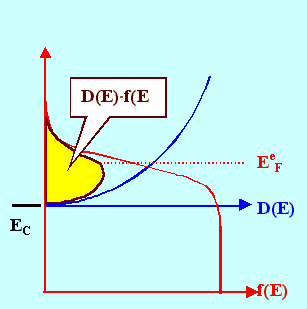Gain CoefficientThe gain coefficient describes how the density of photons, un(z), changes as they propagate along the z-direction. The definition implicitly used before was
un(z)
dz
=  gn · un(z)The physical process for the change of the photon density was stimulated emission (increasing the density) and fundamental absorption (decreasing the density). Both effects we combined into a net emission rate which expresses the balance of emission or absorption rates taking place the photons propagate in z direction:
Rnetse  =  Rse  –  Rfa  =  Rnetse(z)  =  R(z)For the individual emission rates Rse and Rfa we had simplified equations, however, not expressively as a function of z:
Rfa  = Afa · Neff · un · Dn · [1 - fh in V(E v, EFh, T)] · [1  –  fe in C(E c, EFe, T)]

Rse = Ase · Neff · un · Dn · [fe in C(Ec, EFe, T)] · [fh in V(E v, EFh, T)]From a somewhat more detailed look at the inversion condition in an advanced module, using e.g. the proper density of states instead of effective densities, we obtained "better" equations which we are now going to use:
 Rfa(E V, E = Afa  ·  DV(E v) · · DC(E c) · · C) DE v · [1  – DE c · [1  –  f(E v, EFh)]  f(E c, EFe)] æ è ö ø æ è ö ø æ è ö ø æ è ö ø

  Rse(E c, E = Ase  ·  DV(E v) · · DC(E c) · v) DE v · [1  – DE c · f(E  f(E v, EFh)] c, EFe) æ è ö ø æ è ö ø æ è ö ø æ è ö øSumming up (= integration) for all possible transitions gives for Rnet
Rnet ó õ ECThe change in the density of the photons is now directly given by
un(z,t)
t
=  Rnet
which we can write as
un(z,t)
t
=  un(z,t)
z
·   z
t
=  RnetWe use the partial derivative signs to make clear that we have more than one variable.This may look at bit strange. What does it mean?It means that the density of a bunch of photons that are contained in some volume element at some point z is given by the product of the change in density along z that they experience in their travel, times the rate with which they change their position and this means that
¶z ¶t group velocity of the photons .Look at a simple analogy:When you and your friends travel as a group from Kiel to Munich, starting with some amount of money mKiel, which will cerainly change by the time you reach Munich, you have a certain value of the money gradient dm/dl along the length l of your path.Your rate of spending, dm/dt, depends on how much you spent along the way ( = dm/dl) times how fast you spent it ( = dl/dt),
dm
dt
=  dm
dl
·   dl
dtand dl/dt is just the velocity with which you move.For {un(z,t)/z} · {z/t} we already have the independent expression that defined the gain coefficient from above, and we also have the lengthy expression for Rnet. Inserting it yields
Rnet = gn(z) DC(E v  +  · · vg · un = A hn) · DV(E · un v)] ó õ EC æ è ö ø æ è ö øfrom which we obtain the final formula

gn  =  A   ·   DC(E  +  hn) · DV(E V) vg ó õ EC æ è ö ø æ è ö øThis looks complicated (actually, it is complicated) - but it is a clear recipe for calculating g.Essentially, the integral as a function of the frequency n scales with the density of electrons in the conduction band and the density of holes in the valence band exactly hn electron volts below. Both values increase if the Quasi Fermi energies move deeper into the bands.The integral runs over the valence band, summing up all energy couples between the valence band and the conduction band that are separated by hn; it will thus be a function of n. For some n, depending on the carrier concentration, it will have a maximum. This is easy to see if we consider the distribution of electrons (or holes) in the conduction (or valence) band.In this example for the conduction band, the quasi Fermi energy is somewhere above the band edge. The product of the Fermi distribution with the density of states (here as the standard parabola from the free electron gas approximation) always will give a pronounced maximum somewhere between EC and EeF. The same thing happens for the holes in the valence band.The energy difference between the two maxima will be the energy or frequency where gn is largest. If we increase the carrier concentrations, i.e. if we move the quasi Fermi energies deeper into the bands, gn will increase too, and the maximum value shifts to somewhat larger energies.All things considered, we now have:A good idea of how to calculate gn and what we need to know for the task.A good idea of the general behavior of gn and what we have to do in a qualitative way to change its value to what we want.A pretty good grasp why gn looks the way we have drawn it - without justification - in a backbone module.Phase and Group Velocity6.1.2 Light Amplification in Semiconductors6.1.3 Oscillation and Second Laser ConditionDetailed Derivation of the Inversion Condition

© H. Föll (Semiconductors - Script)